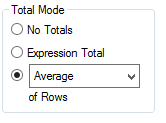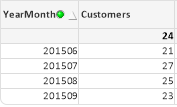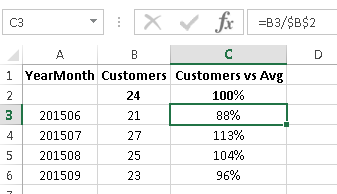# QlikView Creating Analytics

Discussion Board for collaboration related to Creating Analytics for QlikView.Not applicable

## Dividing by the average of a distinct count in a straight table

Hello everyone,

It's very easy to construct the following straight table in Qlikview with the dimension YearMonth, expression count(distinct CustomerID) andI need to add a column which will divide the number of unique customers per month by the average. In Excel this is very simple:How do I create this column in Qlikview?

1 Solution

Accepted SolutionsMVP

## Re: Dividing by the average of a distinct count in a straight table

Hi, you can try with:

count(distinct CustomerID)/Avg(TOTAL Aggr(count(distinct CustomerID), YearMonth))

3 RepliesMVP

## Re: Dividing by the average of a distinct count in a straight table

Hi, you can try with:

count(distinct CustomerID)/Avg(TOTAL Aggr(count(distinct CustomerID), YearMonth))MVP

## Re: Dividing by the average of a distinct count in a straight table

count(DISTINCT Customer)

/

(count(TOTAL DISTINCT Customer)/count(TOTAL DISTINCT YearMonth))Not applicable

## Re: Dividing by the average of a distinct count in a straight table

Thanks Ruben and Maxgro.

count(DISTINCT Customer)

/

(count(TOTAL DISTINCT Customer)/count(TOTAL DISTINCT YearMonth))

only works well with count and sum. Since customers are able to purchase in multiple months, doing a count total distinct will count such customers only once each which means that (count(TOTAL DISTINCT Customer)/count(TOTAL DISTINCT YearMonth)) is not actually equal to the average number of customers per month.

Avg(TOTAL Aggr(count(distinct CustomerID), YearMonth)) takes care of this and appears to be the formula which Qlikview uses in calculation when we tick the box below:- thanks @Ruben!